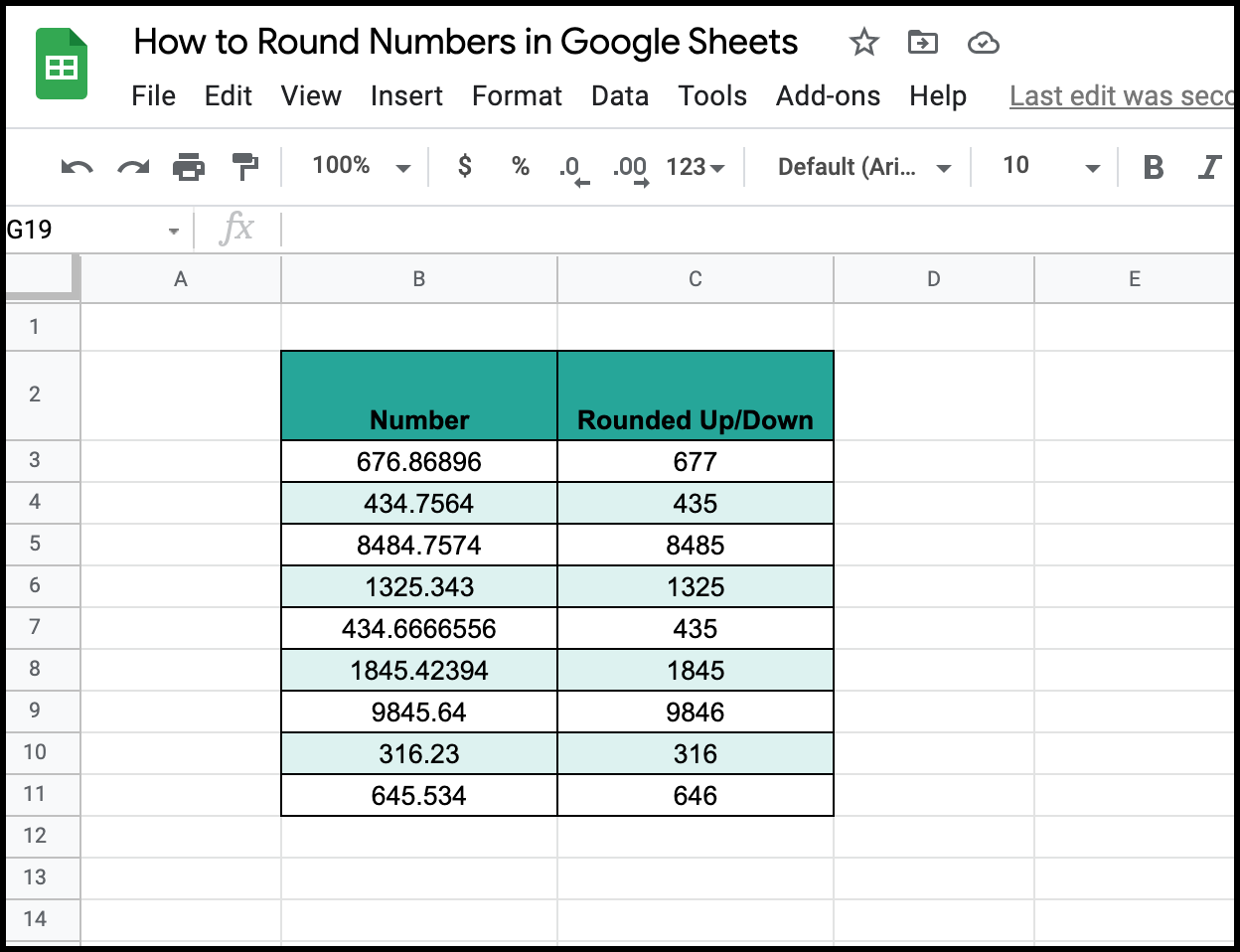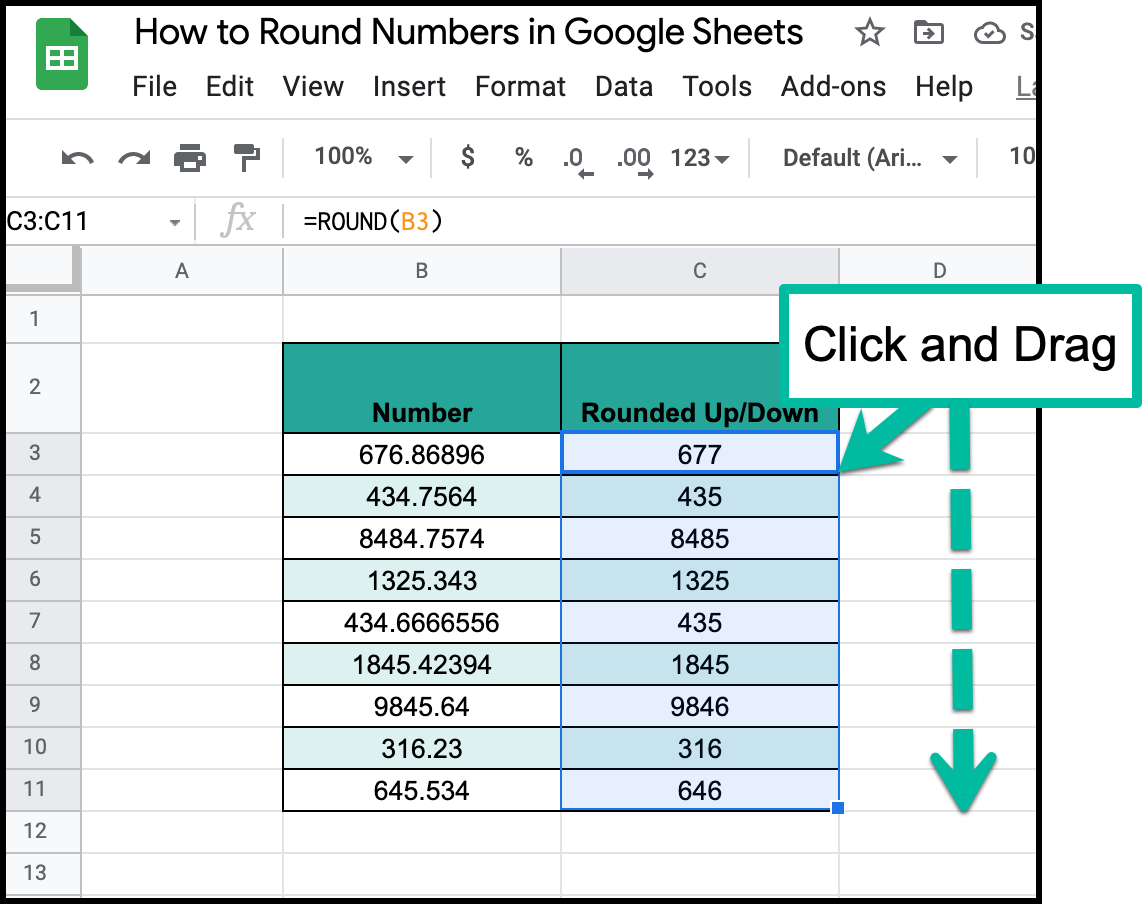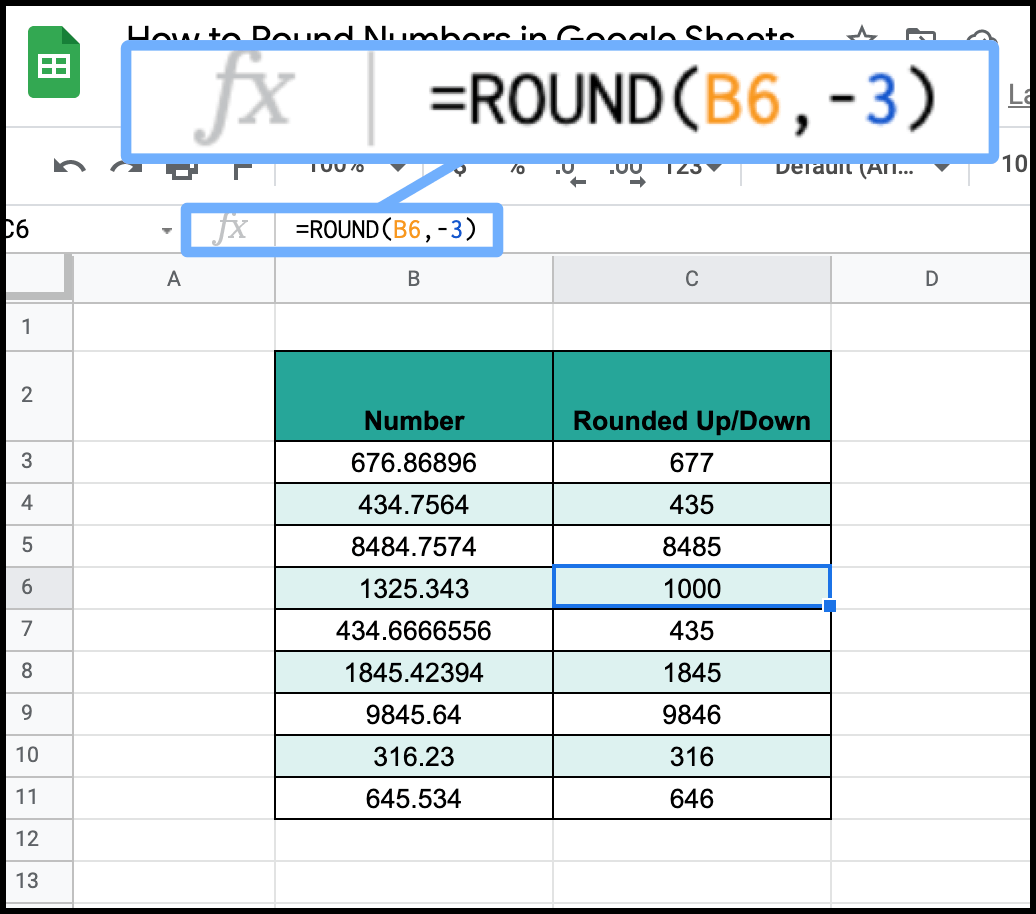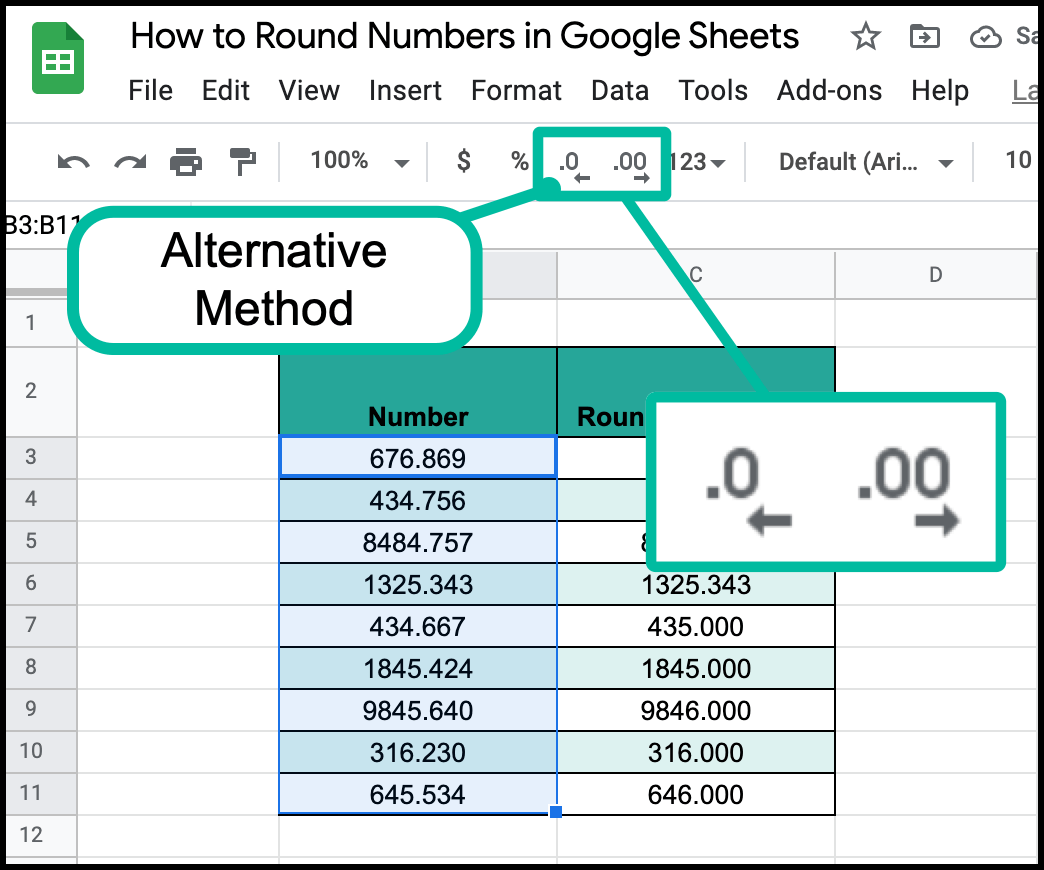# How to Round Numbers in Google SheetsThe ROUND function will let you round your cells to the nearest decimal place of your choosing. Learn how to do so with two methods. You can use these techniques to round your numbers to the nearest decimal places required.

## "=ROUND(Cell)"The first technique is to use the function =ROUND(Cell).

This will round your numbers to the nearest ones place.

From there you can simply click and drag the function throughout all of your numbersFrom there simply click and drag the function throughout the rest of your column.

## "=ROUND(Cell, Tens-Place)"If you feel like getting fancy with it, or you need to round your value to a different decimal, try this formula. You can use it to tell google sheets to how many decimal places you want it rounded.

In the example above, =ROUND (B6, -3) means that you will round 1325.343 to the nearest 10^3 spot, meaning increments of 1000. That being said, the yield of cell C6 will be 1000.

If I wrote =ROUND (B6,2) that would round the number to the nearest hundredths place (10^-2). That would be 1325.34, which is 2 decimal points.

## Tool Ribbonone final honorable mention would be the round up/down tools in the top menu toolbar. the one on the left removes decimals, while the one to to right adds decimals.

It will have the same effect as those previous functions, and I personally prefer it, because it is way quicker and easier than the other two. Also, make sure that you highlight all of the cells that you want to round up or down.

And there you have it; that is how you round numbers in Google Sheets.